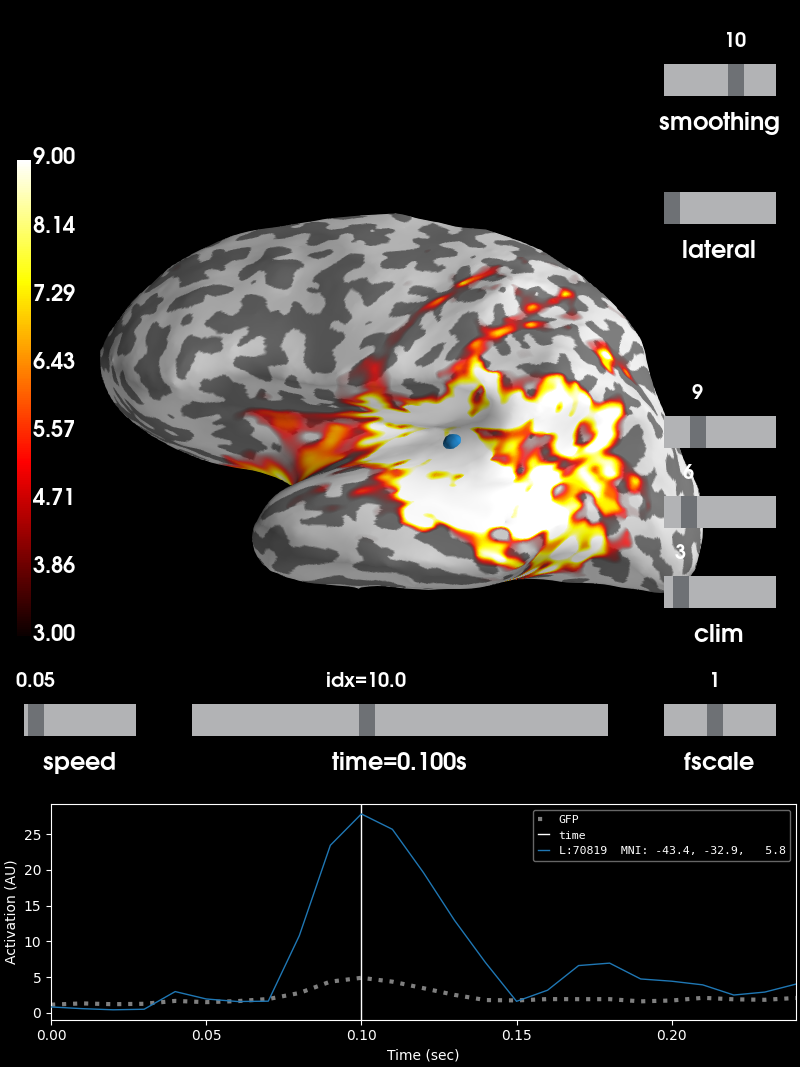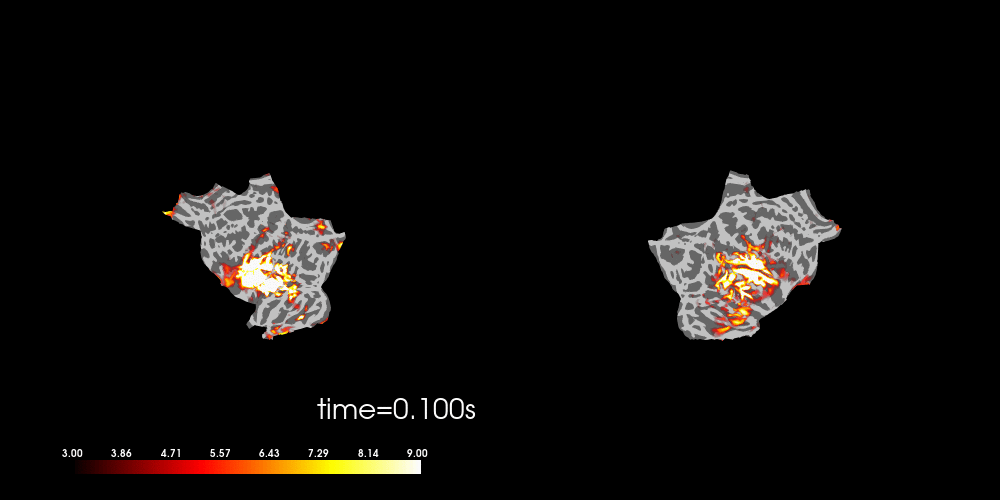# Visualize source time courses (stcs)¶

This tutorial focuses on visualization of stcs.

## Surface Source Estimates¶

First, we get the paths for the evoked data and the time courses (stcs).

import os
import os.path as op

import numpy as np
import matplotlib.pyplot as plt

import mne
from mne.datasets import sample

data_path = sample.data_path()
sample_dir = os.path.join(data_path, 'MEG', 'sample')
subjects_dir = os.path.join(data_path, 'subjects')

fname_evoked = data_path + '/MEG/sample/sample_audvis-ave.fif'
fname_stc = os.path.join(sample_dir, 'sample_audvis-meg')


Then, we read the stc from file

stc = mne.read_source_estimate(fname_stc, subject='sample')


This is a SourceEstimate object

print(stc)


Out:

<SourceEstimate | 7498 vertices, subject : sample, tmin : 0.0 (ms), tmax : 240.0 (ms), tstep : 10.0 (ms), data shape : (7498, 25), ~791 kB>


The SourceEstimate object is in fact a surface source estimate. MNE also supports volume-based source estimates but more on that later.

We can plot the source estimate using the stc.plot just as in other MNE objects. Note that for this visualization to work, you must have mayavi and pysurfer installed on your machine.

initial_time = 0.1
brain = stc.plot(subjects_dir=subjects_dir, initial_time=initial_time,
clim=dict(kind='value', lims=[3, 6, 9]))You can also morph it to fsaverage and visualize it using a flatmap:

stc_fs = mne.compute_source_morph(stc, 'sample', 'fsaverage', subjects_dir,
smooth=5, verbose='error').apply(stc)
brain = stc_fs.plot(subjects_dir=subjects_dir, initial_time=initial_time,
clim=dict(kind='value', lims=[3, 6, 9]),
surface='flat', hemi='split', size=(1000, 500),
smoothing_steps=5, time_viewer=False,